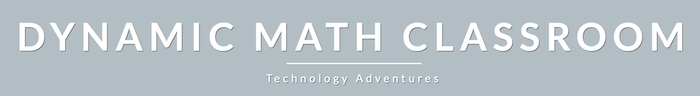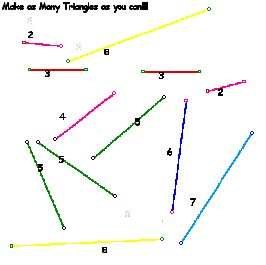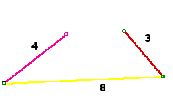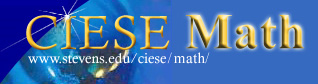Lesson: Tris and Untris
 Student Teacher Extensions
Part 1 - Making Triangles

Below are 12 line segments (or straws) of various length. Make as many unique triangles as you can using these segments. Use this Sketchpad file to help you.After you make your triangles, record them in the table below.

 length A length B length C What kind of triangle is it? 1 2 3 4 5 6

Part 2 - Making “Untriangles”

We know that a triangle has three sides that form a closed shape. But what’s an "untriangle?"

Below is an example of an untriangle. Why do you think it's called an untriangle?Make and record your untriangles below:

 length A length B length C Why is this an untriangle? 1 2 3 4

Below are candidates for “trianglehood.” Indicate which are triangles and which are “wannabe” triangles (untriangles).

 length A length B length C Triangle (T) or untriangle (U)? Explain why T or U? a 2 3 4 b 8 4 2 c 6 6 6 d 7 4 3 e 3 6 3 f 8 6 6 g 2 3 2 h 2 5 2 i 8 7 6 j 3 4 5

Some Questions:

1. If two of the line segments are equal, will you always be able to make a triangle with the third stick? Explain.

2.
Can you always make a triangle if all three segments are equal? If so, what is this triangle called?

3. Explain when it is possible for you to make a triangle with three segments.

4. Explain when it is NOT possible for you to make a triangle with three segments.Source: Original Version 1.0 developed at CIESE
Center for Innovation in Engineering & Science Education (2007)
Revised 11.13.19 currently under construction